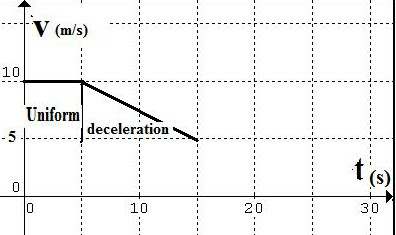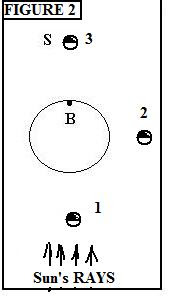## Tuesday, May 10, 2011

### Previous Mechanics Problems solvedWe now look at solutions to previous problems from the Basic Physics series:

From Mechanics, Pt. 2:

Problems:

1)A car moves at 10 m/s for a period of 5 seconds then slows to 5 m/s over the next 10 seconds. Explain the changes in motion and which, if any, of Newton’s laws apply during the stages of the motion, giving reasons.

The graph attached shows the changes, with the motion of the first five seconds labeled “uniform” – for uniform velocity (so Newton’s 1st law applies) and the next segment of motion for 10 secs labeled ‘deceleration’ since the velocity shows a braking from 10 m/s to 5 m/s over 10 seconds, for a deceleration of:

a= (10 – 5)m/s/ 10 s = 5m/s/ 10 s = 0.5 m/s^2

Thus Newton’s 2nd law applies owing to the presence of a resultant (e.g. braking) force

2)For the demonstration of Newton’s 1st law in Fig.1 (previous instalment), let f = u_k N, where N is the normal force and u_k is the coefficient of kinetic friction. If the weight of the mass being moved is 1 kg and u_k = 0.1, what is the smallest magnitude of the applied force F needed to still allow the 1st law to apply?

The smallest applied force is that applied force F that is just adequate to overcome the frictional force f, to obtain a net resultant force (F – f) > 0.

For m = 1 kg, mg = 1kg(10 m/s^2) = 10 N

N = 10N

So f = 0.1(10N) = 1N

Then, within the limits of measurement (for a dynamometer that reads N to increments of 0.1N), F = 1.1N

From Mechanics, Pt. 5:

Problems:

1(a) Based on the diagram provided (lower graphic), explain how it is that S remains stationary to an observer B but would not appear stationary to an observer on the Sun.

The reason that S remains stationary relative to observer B (on Earth) is that the velocity of S is identical to the Earth’s rate of rotation, and hence S appears to be fixed in relation to B. To an observer on the Sun this doesn’t apply since the rate of rotation of the Sun (~ 26 days) would have no relation to the rate of the satellite S over Earth. (Once every 24 hours)

b)What is the time for S to make two orbits of the Earth? Justify your answer.

The time would be twice the geosychronous period. Since one orbit takes one day (being equal to Earth’s rate of rotation) then two orbits would take two days.

c)To an observer on S, would the Earth show phases, as the Moon does to observers on Earth? Illustrate your answer with a diagram.

Yes, similar phases would be displayed as shown in the diagram (Fig. 2). As can be seen at position (1) with Sun’s rays in the specified direction, S would be in the “New” phase, at position (2) it would display what we’d term “first quarter” on Earth, and in position (3) the full phase since the whole half of S facing Earth is now illuminated.

d)Imagine the distance between S and the Earth’s center is increased. What effect, if any, would this have on the period?

The period would be increased

e)Assuming the specific increased distance D = 2 R (where R = d + r is the original distance, and r is the Earth’s radius) what is the effect on the force of gravitational attraction exerted by Earth on S? Comment on the statement: “S has mass, but no weight”.

If D = 2R then, and F_G ~ 1/D^2, and g ~ 1/r^2

But: at r we have g (= 9.8 m/s^2). At R = d + r we have R = 6.6r + r = 7.6 r, so g' = g/(7.6)^2. Increasing to D = 2R = 2(d +r) = 2(6.6r + r) = 2(7.6r) = 15.2 r, then g"= g/((15.2)^2. They key point is that g decreases as the square of the distance and one needs to use g referenced to Earth's surface (r) to find any new g-value (g', g" etc.) Thus the first order of business is to note that 42 400 km = 6.6r when r = 6 400km.

2. a) Given that Jupiter's value for a = 5.2 AU, determine the length of its year.

Use Kepler’s harmonic law, then:

(P1/ P2)^2 = (a1/ a2)^3

We take P2 = 1 year (the period for Earth to make one revolution).

We take a2 = 1 AU or astronomical unit

a1 (Jupiter) = 5.2 A.U.

Then (P1)^2 = [(P2)^2 (a1/ a2)^3] =

P1 = [(1 yr.)^2(5.2 A.U./ 1 A.U.)^3]^ ½ = [140.6]^ ½ = 11.8 yrs.

b) Given that Jupiter's eccentricity e = 0.048 find the displacement distance D from its orbital ellipse center.

We have: D = e x a = (0.048) x (5.2 A.U.) = 0.25 A.U. = 3.75 x 10^10 m

Mechanics – Pt.6 (last two parts of the problem)

b)Show by Newton’s 3rd law that on collision, the forces exerted by the masses are equal and opposite.

We require F12 = -F12

The masses are given as the same (m1= m2) then the velocities must be found to be equal and opposite, i.e. the relative velocity of the masses before collision must equal to the relative velocity after.

Since the conditions denote a perfectly elastic collision (frictionless surface), then both (1) conservation of linear momentum and (2) conservation of kinetic energy are satisfied, hence:

(1) m1u1 + m2u2 = m1v1 + m2v2

(2) ½ m1u1^2 + ½ m2u2^2 = ½ m1v1^2 + ½ m2v2^2

Cancel through the factors ½ on each side and get:

m1u1^2 + m2u2^2 = m1v1^2 + m2v2^2

Transpose and rewrite:

= m1(u1^2 – v1^2) = m2(v2^2 – u2^2)

Factor both sides:

-> m1(u1 – v1)(u1 + v1) = m2(v2 – u2)(v2 + u2)

Go back to conservation of momentum eqn. and separate terms:

-> m1(u1 – v1) = m2(v2 – u2)

Divide the (factored) energy equation by this one for the linear momentum, and insert correct vector designations (moving right is +ve, moving left is –ve):

(u1 – u2) = -(v1 – v2)

Thus the relative velocities of the two bodies before collision is equal to the negative of the relative velocities after collision. Since the masses are equal and the collision occurs in the same time delta t, then the forces must be equal and opposite.

c)Hence or otherwise, show the total momentum before the collision is the same as the total momentum after collision (take the momentum to be the product of the mass times the velocity).

We first not that v1 and v2 can be obtained from the last equation for relative velocities before and after collision. Thus:

v2 = (u1 – u2) + v1

v1 = (u2 – u1) + v2

We can then use the original two equations for conservation of linear momentum and conservation of kinetic energy to separately find:

v1 = [(m1 – m2)/ (m1 + m2)] u1 + [2m2/ (m1 + m2)] u2

v2 = [2m1/(m1 + m2)]u1 + [(m2 – m1)/ (m1 + m2)]u2

Now, if m1 = m2 then we can simplify to get(inserting correct vector directions):

v1 = [2m2/ (m1 + m2)](- u2) = -(2m/2m)u2 = -u2

v2 = = [2m1/(m1 + m2)]u1 = (2m/2m) u1 = u1

Then, momentum before collision: m1u1 – m2u2 = mu1 – mu2

And after collision: m1(-u2) + m2(u1) = mu1 – mu2

Hence, the two are equal. (Note: the procedure should be to work out the velocities v1, v2 with separate masses m1, m2 included, to be able to generalize the result to other elastic collisions which may not have m1 equal m2!)